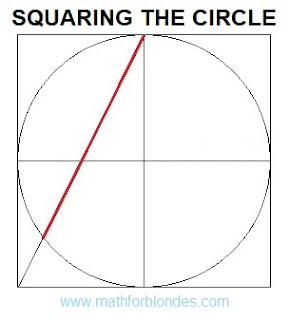## 1/29/2022

### Squaring the circle

>Squaring the circle. The solution of the problem. Almost accurate. Math draw.Squaring the circle. The solution of the problem. Almost accurate.

This construction is not a solution to the problem of squaring the circle, but it forced me to get my hands on the math and do all the verification calculations. If the correct solution should give a segment with a length of 1.77245 radii, then the length of the red segment in the figure is 1.78885 radii. A little more than necessary. How much more? The segment exceeds the required length by 0.9253% or by 0.01640 of the radius. The area of a square with sides equal to the length of this segment is 1.8592% greater than the area of a circle.

How to use it? For a friendly prank of math lovers. Build a square with sides equal to ten centimeters. Inscribe in a square a circle with a radius of 5 centimeters. Draw a line as shown in the figure and offer a ruler to measure the length of the resulting segment. It will be equal to 8.9 centimeters. Using a calculator, calculate the area of a circle, which is equal to 78.54 square centimeters. The area of a square with sides of 8.9 centimeters is 79.21 square centimeters. This discrepancy can be explained by the low accuracy of measurements using a ruler. Personally, after such rough calculations, I sat down to check the solution.

I don't recommend this kind of joke with my teachers or math teachers - they can force you to do all the verification calculations to refute this solution. The unsolvability of this problem was proved by the German mathematician Lindemann back in 1882. However, I have long been very distrustful of many proofs of mathematicians.